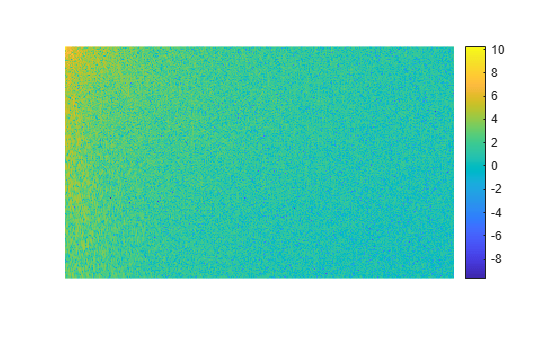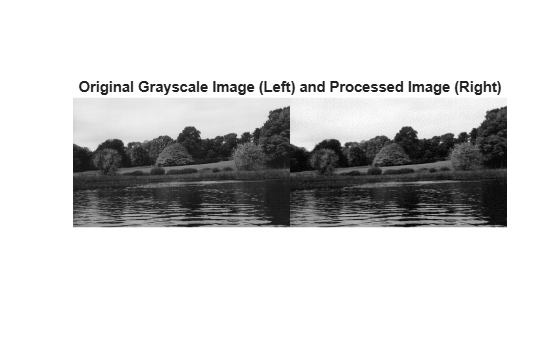# idct2

2-D inverse discrete cosine transform

## Syntax

``B = idct2(A)``
``B = idct2(A,m,n)``
``B = idct2(A,[m n])``

## Description

example

````B = idct2(A)` returns the two-dimensional inverse discrete cosine transform (DCT) of `A`.```
````B = idct2(A,m,n)` and `B = idct2(A,[m n])` pads `A` with `0`s to size `m`-by-`n` before applying the inverse transformation. If `m` or `n` is smaller than the corresponding dimension of `A`, then `idct2` crops `A` before the transformation.```

## Examples

collapse all

This example shows how to remove high frequencies from an image using the two-dimensional discrete cosine transfer (DCT).

Read an image into the workspace, then convert the image to grayscale.

```RGB = imread('autumn.tif'); I = rgb2gray(RGB);```

Perform a 2-D DCT of the grayscale image using the `dct2` function.

`J = dct2(I);`

Display the transformed image using a logarithmic scale. Notice that most of the energy is in the upper left corner.

```figure imshow(log(abs(J)),[]) colormap(gca,jet(64)) colorbar```Set values less than magnitude 10 in the DCT matrix to zero.

`J(abs(J) < 10) = 0;`

Reconstruct the image using the inverse DCT function `idct2`.

`K = idct2(J);`

Display the original grayscale image alongside the processed image.

```figure imshowpair(I,K,'montage') title('Original Grayscale Image (Left) and Processed Image (Right)');```## Input Arguments

collapse all

Input matrix, specified as a 2-D numeric matrix.

Data Types: `single` | `double` | `int8` | `int16` | `int32` | `int64` | `uint8` | `uint16` | `uint32` | `uint64`

Number of image rows, specified as a positive integer. `idct2` pads image `A` with `0`s or truncates image `A` so that it has `m` rows. By default, `m` is equal to `size(A,1)`.

Number of image columns, specified as a positive integer. `idct2` pads image `A` with `0`s or truncates image `A` so that it has `n` columns. By default, `n` is equal to `size(A,2)`

## Output Arguments

collapse all

Transformed matrix using a two-dimensional discrete cosine transform, returned as an `m`-by-`n` numeric matrix.

Data Types: `double`

## Tips

• For any matrix `A`, `idct2(dct2(A))` equals `A` to within round-off error.

## Algorithms

`idct2` computes the two-dimensional inverse DCT using:

where

and

 Jain, A. K., Fundamentals of Digital Image Processing, Englewood Cliffs, NJ, Prentice Hall, 1989, pp. 150-153.

 Pennebaker, W. B., and J. L. Mitchell, JPEG: Still Image Data Compression Standard, New York, Van Nostrand Reinhold, 1993.

﻿